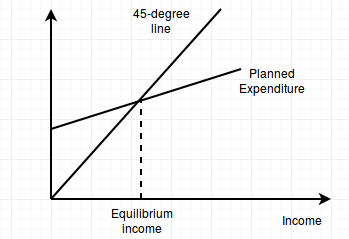Consider an economy with I = 0, G = 0, T = 0, and NX = 0, but with the following consumption...

Question:

Consider an economy with I = 0, G = 0, T = 0, and NX = 0, but with the following consumption function:

{eq}C = \bar{C} + MPC * Y {/eq}.

a) Draw a graph showing the equilibrium level of output.

b) How would your graph change if there was an increase in the MPC?

Keynesian Cross Model:

The Keynesian cross model is a model of short-run income in the Keynesian school of thoughts. The model characterizes income in the short-run as the equalization between planned expenditures and actual expenditures. In this model, fiscal policies have a large effect on short-run income.

a) The following diagram shows the determination of equilibrium income in the short run.b) If the marginal propensity to consume increases,...

Become a Study.com member to unlock this answer! Create your account